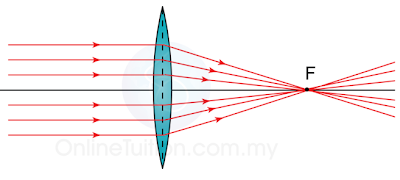# Power of Lenses

### The Power of a Lens

1. The power of a lens is defined as the reciprocal of the focal length in unit meter. $P= 1 f$ Important Note: f is in meter
2. The unit of power is diopter (D).
3. The relationship of the power with the thickness and types of lens are shown in the diagram below.
 Lens Power of the Lens Converging (Convex) Positive Diverging (Concave) Negative Thick, with short focal length. High Thin, with long focal length. LowThinner – Lower Power – Longer Focal LengthThicker – Higher Power – Shorter Focal Length

Example:
The power of a lens is labeled as +5D. What is the focal length of the lens (in cm)? Is this a concave lens or a convex lens?